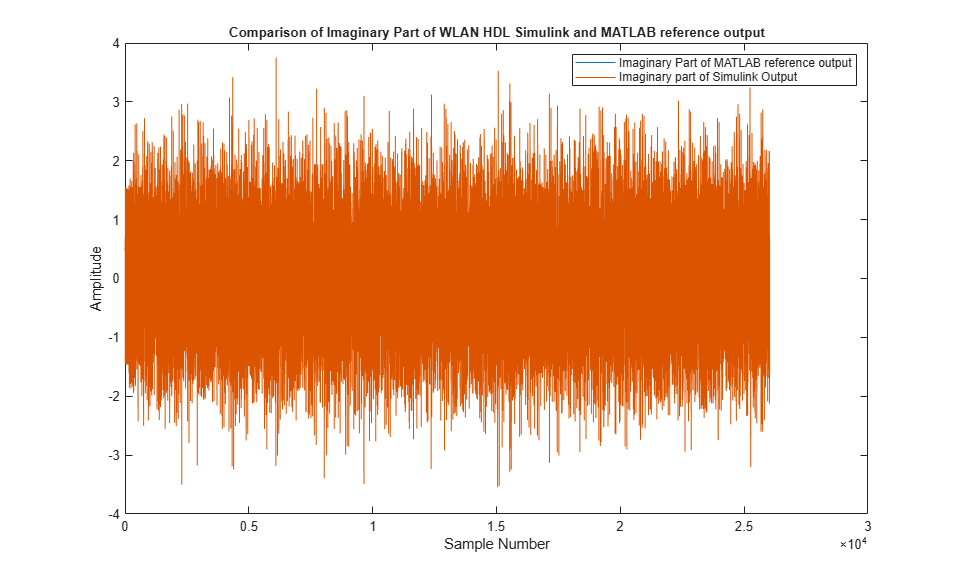# WLAN HDL Time and Frequency Synchronization

This example shows how to implement a WLAN time and frequency synchronization model that is optimized for HDL code generation and hardware implementation. Time and frequency synchronization are the key steps to recover wireless local area network (WLAN) packet information.

The model estimates and corrects the time and frequency offsets in the received WLAN signal that are introduced by wireless channel and radio frequency (RF) front-end impairments. Initially, the model performs coarse time and frequency estimation and corrections on the received signal. Then, the model fine tunes the time and frequency estimation and corrections on the received signal to remove any residual offsets. The model supports 20, 40, and 80 MHz bandwidth options for non-high throughput (Non-HT), high throughput (HT), very high throughput (VHT), and high efficiency (HE) frame formats. The example compares the Simulink® model output with the MATLAB® functions by using WLAN Toolbox™ features.

WLAN packet decoding includes these stages: time and frequency synchronization, OFDM demodulation, channel estimation & equalization, format detection, signal decoding, and data decoding.In this decoding procedure, only the time and frequency synchronization stage can be optimized for HDL code generation. The HDL support is extended for other stages in a future release.

In MATLAB, run this command to open the example model.

```model_name = 'wlanhdlTimeAndFrequencySynchronization'; open_system(model_name); ```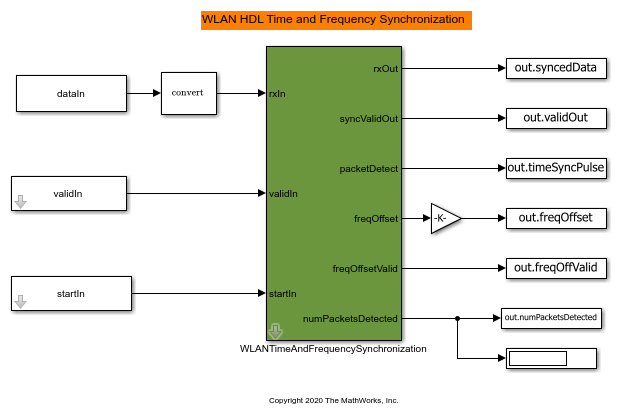The `WLANTimeAndFrequencySynchronization` model contains these subsystems: Coarse Time Sync, Coarse CFO Estimation and Correction, Fine Time Sync, and Fine CFO Estimation and Correction.

In MATLAB, run this command to open the WLANTimeAndFrequencySynchronization subsystem.

```open_system([model_name '/WLANTimeAndFrequencySynchronization'],'force'); ```### Coarse Time Synchronization

The coarse time synchronization algorithm implements a double sliding window for correlation as described in the MATLAB function `wlanPacketDetect.m`. The Coarse Time Sync subsystem uses the autocorrelation of legacy short training field (L-STF) symbols to return an estimated packet-start offset. The Peak Detector subsystem compares the correlation metrics with the energy of the signals and determines the start of the packet. In the next stage, the fine symbol timing detection refines this packet start estimate using the legacy long training field (L-LTF).

In MATLAB, run this command to open the Coarse Time Sync subsystem.

```open_system([model_name '/WLANTimeAndFrequencySynchronization/Coarse Time Sync']); ```### Coarse CFO Estimation and Correction

Considering the start of the packet from the Coarse Time Sync subsystem, Coarse CFO Estimation and Correction subsystem performs autocorrelation on the input using a L-STF and averages the calculated correlation metrics over a window of the L-STF duration. Then, the subsystem estimates the carrier frequency offset (CFO) by considering the angle of the resulted metric.

In MATLAB, run this command to open the Coarse CFO Estimation subsystem.

```open_system([model_name '/WLANTimeAndFrequencySynchronization/Coarse CFO Estimation and Correction/Coarse CFO Estimation']); ```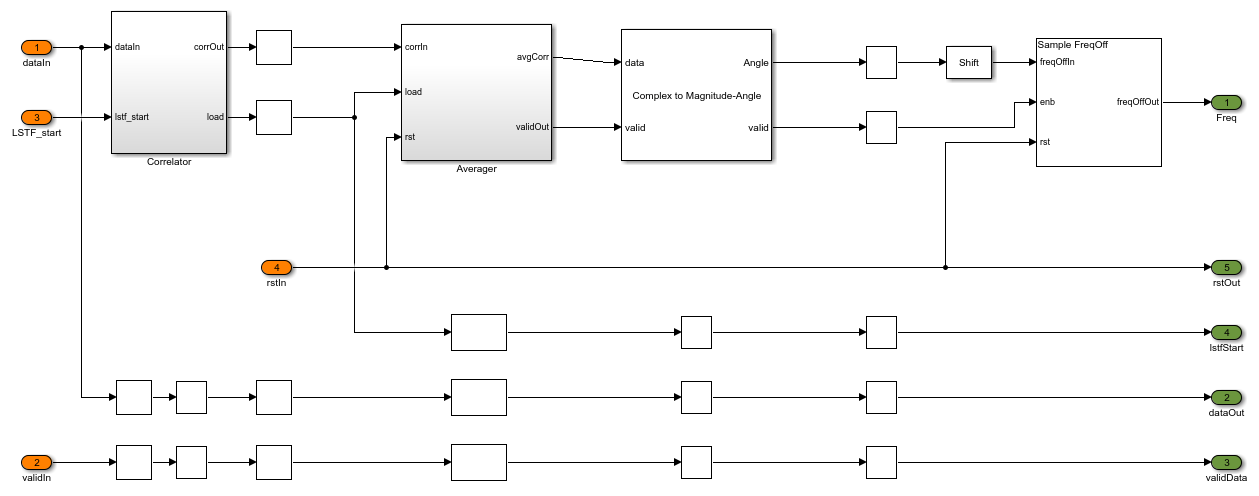This subsystem uses the CFO estimate to correct the frequency offset.

In MATLAB, run this command to open the Coarse CFO Correction subsystem.

```open_system([model_name '/WLANTimeAndFrequencySynchronization/Coarse CFO Estimation and Correction/Coarse CFO Correction']); ```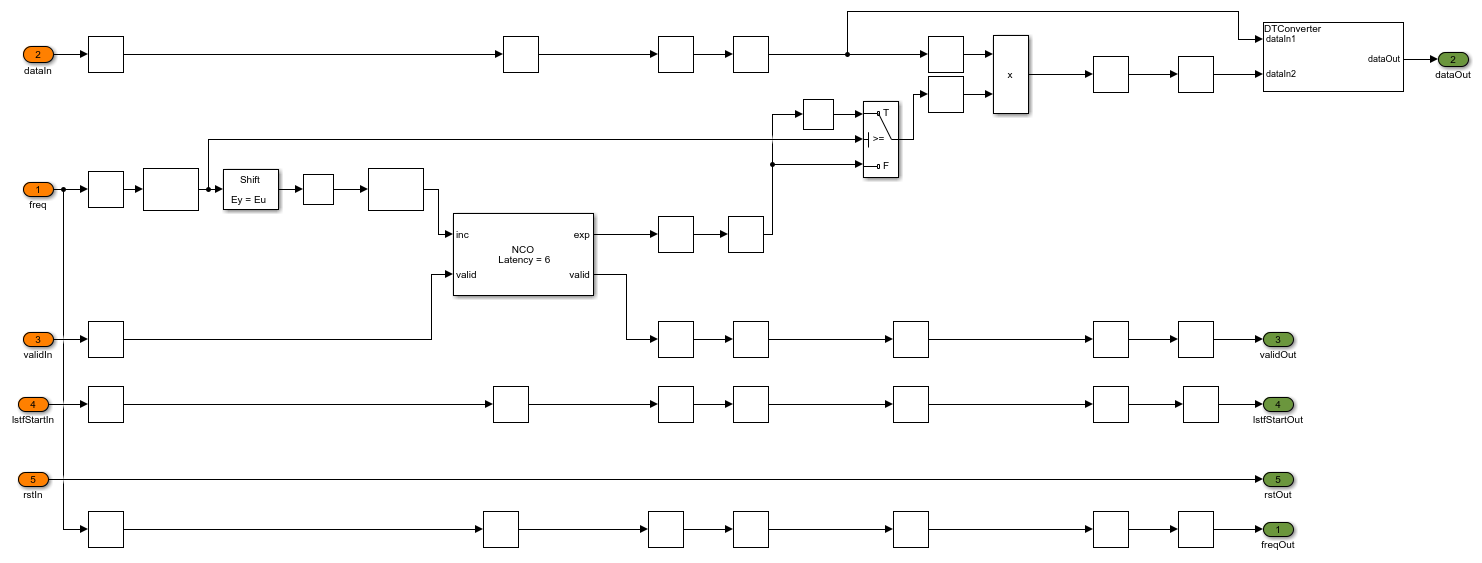### Fine Time Synchronization

The Fine Time Sync subsystem takes the coarsely corrected time and frequency offset waveform for fine time offset synchronization. The Correlator subsystem cross correlates the received signal with the locally generated L-LTF. The Peak Searcher subsystem searches the maximum correlation peak and then synchronizes the signal.

In MATLAB, run this command to open the Fine Time Sync subsystem.

```open_system([model_name '/WLANTimeAndFrequencySynchronization/Fine Time Sync']); ```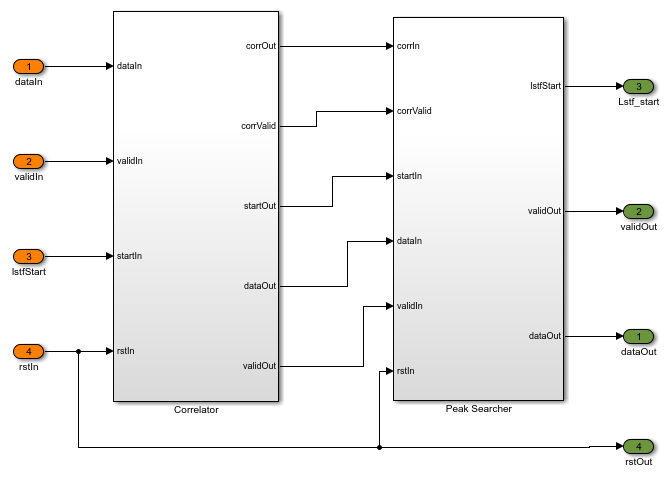### Fine CFO Estimation and Correction

The Fine CFO Estimation and Correction subsystem takes a fine time synced waveform as an input for fine tuning the frequency offset. This subsystem estimates and corrects CFO to remove any residue left after coarse frequency correction, performs fine CFO estimation similar to coarse estimation by using the L-LTF instead of the L-STF, and estimates the frequency offset by considering the angle of the averaged correlations.

In MATLAB, run this command to open the Fine CFO Estimation subsystem.

```open_system([model_name '/WLANTimeAndFrequencySynchronization/Fine CFO Estimation and Correction/Fine CFO Estimation']); ```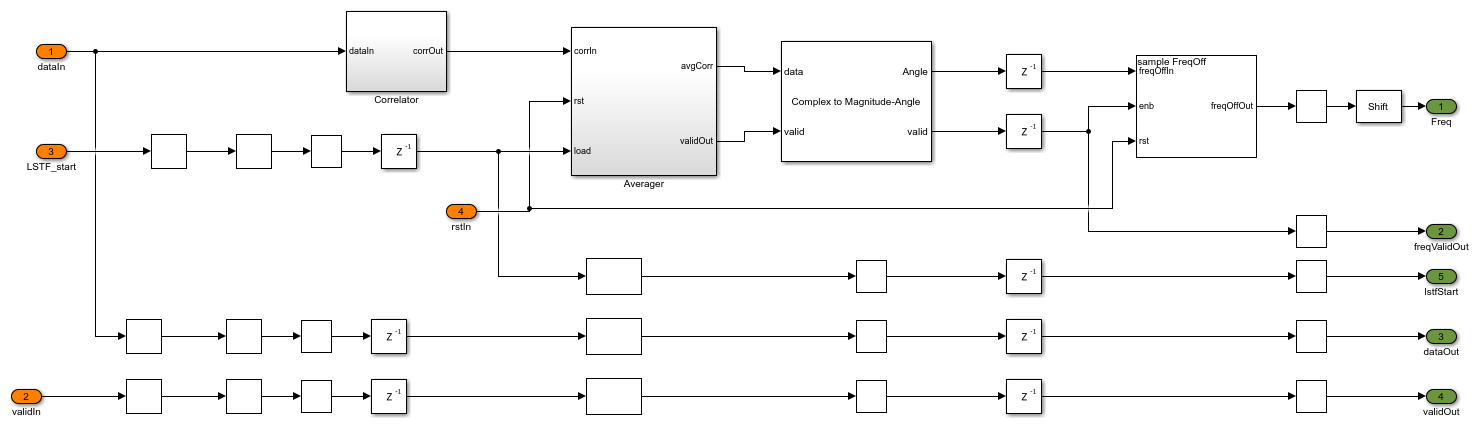The Fine CFO Correction subsystem uses the estimated fine CFO for correcting the residual frequency offset and then outputs the corrected WLAN received signal.

In MATLAB, run this command to open the Fine CFO Correction subsystem.

```open_system([model_name '/WLANTimeAndFrequencySynchronization/Fine CFO Estimation and Correction/Fine CFO Correction']); ```### Model Interface and Verification

The example model accepts the received waveform as an input along with valid and start signals. The model returns a synchronized waveform as an output along with a valid signal. The other outputs in the example include a packet detected flag, a CFO estimate along with its valid and the number of packets detected as an output. CFO estimate is the sum of coarse CFO and fine CFO estimates. The `wlanFrontEndInit` script provides the input to the model. The `wlanWaveformGenerator.m` function in the script generates the VHT 20 MHz frame, which is passed through the TGac channel with a delay profile of Model A. The additive white Gaussian noise (AWGN) at 30 dB signal-to-noise ratio (SNR) is added with other channel impairments of a 10 kHz CFO and a timing offset of '25'.

```fprintf('\n Simulating HDL time and frequency synchronization \n'); out = sim('wlanhdlTimeAndFrequencySynchronization.slx'); fprintf('\n HDL simulation complete. %d packet detected.',out.numPacketsDetected(end)); ```
``` Simulating HDL time and frequency synchronization HDL simulation complete. 1 packet detected.```

The outputs of example are verified by using WLAN Toolbox functions. Specify the same input waveform for the Simulink model and its MATLAB equivalent function and then compare outputs.

```fprintf('\n Comparing WLAN MATLAB time and frequency synchronization \n') inputWaveformRef = inputWaveform(1:end-length(Hd.Numerator)+1); inputWaveformRef = filter(Hd.Numerator,1,inputWaveformRef); % WLAN packet detection [startOffset,Mn]=wlanPacketDetect(inputWaveformRef,CBW); rxWave1 = inputWaveformRef(startOffset+1:end); % Coarse CFO estimation and correction coarseFreqOff = wlanCoarseCFOEstimate(rxWave1,CBW); rxWave2 = hwlanFrequencyOffsetCorrect(rxWave1,fs,coarseFreqOff); % Fine time synchronization searchBufferLLTF = rxWave2(1:wlanConfig.lstfLen*10+wlanConfig.lltfLen*3); [offset,MN] = wlanSymbolTimingEstimate(searchBufferLLTF,CBW); rxWave3 = rxWave2(offset+1:end); % Fine CFO estimation and correction LTFs = rxWave3(10*wlanConfig.lstfLen+(1:wlanConfig.lltfLen*2)); fineFreqOff = wlanFineCFOEstimate(LTFs,CBW); matOut = hwlanFrequencyOffsetCorrect(rxWave3,fs,fineFreqOff); fprintf('\n MATLAB simulation complete. \n'); simData = out.syncedData; simValid = out.validOut; simOut = double(simData(simValid)); ```
``` Comparing WLAN MATLAB time and frequency synchronization MATLAB simulation complete. ```

### Simulation Results

The example synchronizes the time and frequency of the input waveform generated using the `wlanFrontEndInit.m` script and outputs the time and frequency corrected waveform as shown in this timing diagram.The timing diagram shows that the output `rxOut` is synchronized at the start of the L-STF and that the estimated frequency offset is 9.695 kHz, which is close to the introduced frequency offset of 10 kHz.

### Comparison of Simulink Output and MATLAB Reference Output

```plot(real(matOut)); hold on; simOut = simOut(1:length(matOut)); plot(real(simOut)); title('Comparison of Real Part of WLAN HDL Simulink and MATLAB reference output','FontSize', 10); xlabel('Sample Number'); ylabel('Amplitude'); legend('Real Part of MATLAB reference output','Real part of Simulink output'); figure; plot(imag(matOut)); hold on; simOut = simOut(1:length(matOut)); plot(imag(simOut)); title('Comparison of Imaginary Part of WLAN HDL Simulink and MATLAB reference output','FontSize', 10); xlabel('Sample Number'); ylabel('Amplitude'); legend('Imaginary Part of MATLAB reference output','Imaginary part of Simulink Output'); ```